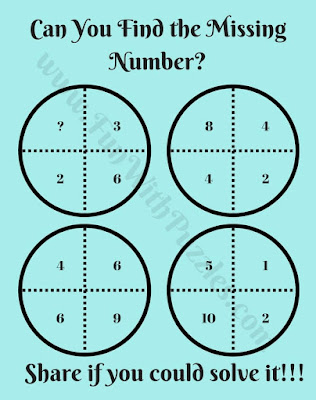Its time to test your Mathematical skills with the Brain Test Math Puzzle. In this puzzle, there are four Circles and each Circle contains four numbers. These numbers in each of the Circles are related to each other by some Mathematical pattern. Can you find this Mathematical pattern and then find the value of missing number which will replace the question mark?Can you find the value of the missing number?

The answer to this "Brain Test Math Puzzle for Teens", can be viewed by clicking on the button. Please do give your best try before looking at the answer.

Unknown said...

1

doctordownvote said...

1

Unknown said...